# Physics Quiz

3.5
10 questions
English
Everyday Physics
1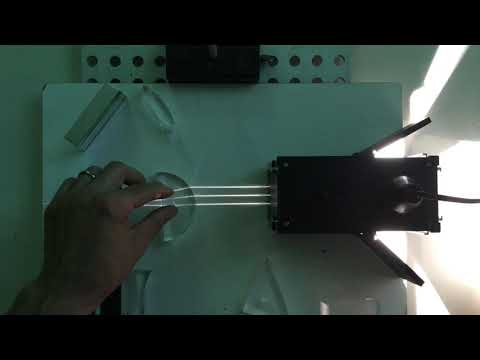## What phenomenon is explained in the Video?

Reflection

Critical angle

Diffraction

Refraction

### 60% got this right

The return of light or sound waves from a surface is called reflection
2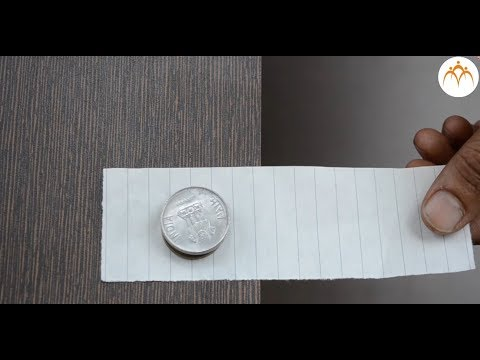## Why did the coin not come along the paper?

Due to Inertia

Coin is not linked with Paper

Coin is too heavy

There is magnet beneath table

### 53% got this right

Objects at rest, tends to remain at rest is called inertia
3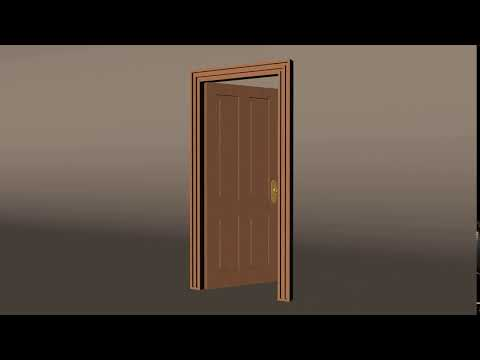## Under what phenomenon does the door open?

Torque

Force

Push

Circular motion

###17% got this right

Torque is a measure of the force that can cause an object to rotate about an axis
4## Which of the following is neither a scalar nor a vector?

Dielectric constant

Angular Momentum

Force

Torque

###32% got this right

These quantizes don't follow law of vector addition. Dielectric is a tensor quantity
5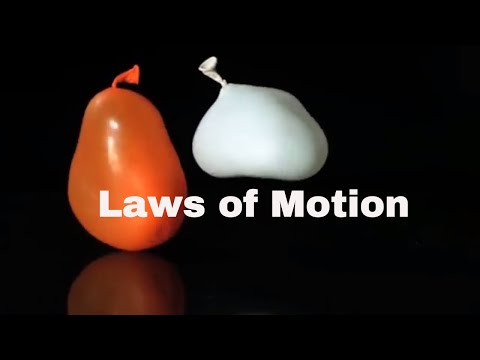## What does Newton's first law tell us?

The definition of force

The forces always exist in pairs

The measure of force

The total quantity of motion

### 36% got this right

First law of motion gives the definition of force.
6## What type of force is non-conservative?

Frictional Force

Gravitational Force

Electromagnetic Forces

Strong Force

###34% got this right

Friction is non-conservative because the amount of work done by friction depends on the path
7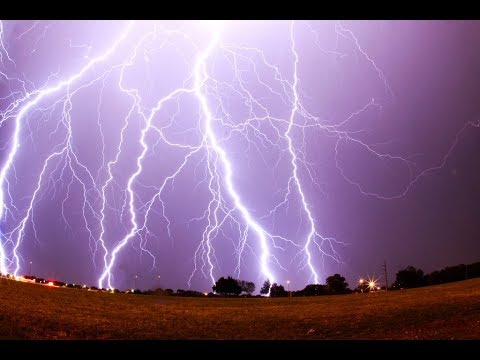## How much energy is in a thunderbolt of lightning?

One billion joules

250000 joules

3000 Joules

500 Joules

### 46% got this right

An average bolt of lightning, striking from cloud to ground, contains roughly one billion joules of eneregy
8## Why does the sea level rise at night?

Moon's gravity

Global Warming

Sea breezes

Sun's gravity

###89% got this right

The sea level rises at night because of the influence of the moon's gravity
9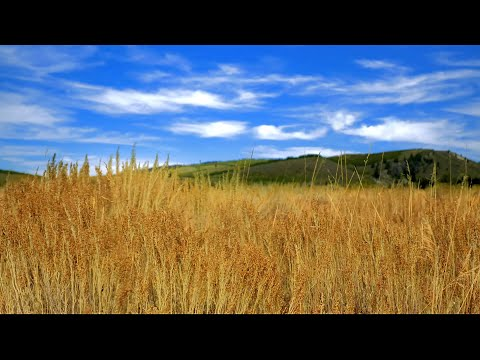## Why are there winds?

Temperature differences

Air flows from high density to low density.

Air flows along isobars

Air flows down-hill

### 36% got this right

The temperature difference at different areas of land cause change in atmospheric pressure. Hence Winds flow
10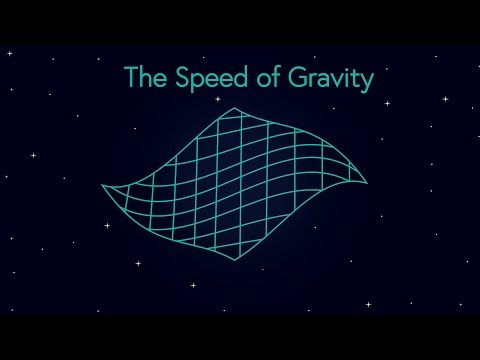## What is the speed of gravity?

Gravity travels at the speed of light

(32 feet) per second per second

Gravity travels at 9.8 meters per second per second

Gravity travels at twice of speed of light

###19% got this right

According to Einstein's General Relativity, gravity travels at the speed of light.

## Similar quizzes3.62.83.6